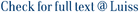We find the minimax rate of convergence in Hausdorff distance for estimating a manifold M of dimension d embedded in R-D given a noisy sample from the manifold. Under certain conditions, we show that the optimal rate of convergence is n(-2/(2+d)). Thus, the minimax rate depends only on the dimension of the manifold, not on the dimension of the space in which M is embedded.

Minimax Manifold Estimation / Genovese, C. R.; Perone Pacifico, Marco; Verdinelli, Isabella; Wasserman, L.. - In: JOURNAL OF MACHINE LEARNING RESEARCH. - ISSN 1532-4435. - 13:(2012), pp. 1263-1291.

### Minimax Manifold Estimation

#### Abstract

We find the minimax rate of convergence in Hausdorff distance for estimating a manifold M of dimension d embedded in R-D given a noisy sample from the manifold. Under certain conditions, we show that the optimal rate of convergence is n(-2/(2+d)). Thus, the minimax rate depends only on the dimension of the manifold, not on the dimension of the space in which M is embedded.
##### Scheda breve Scheda completa Scheda completa (DC)manifold learning; minimax estimation
Minimax Manifold Estimation / Genovese, C. R.; Perone Pacifico, Marco; Verdinelli, Isabella; Wasserman, L.. - In: JOURNAL OF MACHINE LEARNING RESEARCH. - ISSN 1532-4435. - 13:(2012), pp. 1263-1291.
File in questo prodotto:
File
2012_JMLR.pdf

Open Access

Tipologia: Versione dell'editore
Licenza: DRM non definito
Dimensione 2.27 MB
Utilizza questo identificativo per citare o creare un link a questo documento: `https://hdl.handle.net/11385/182608`
•64
•58